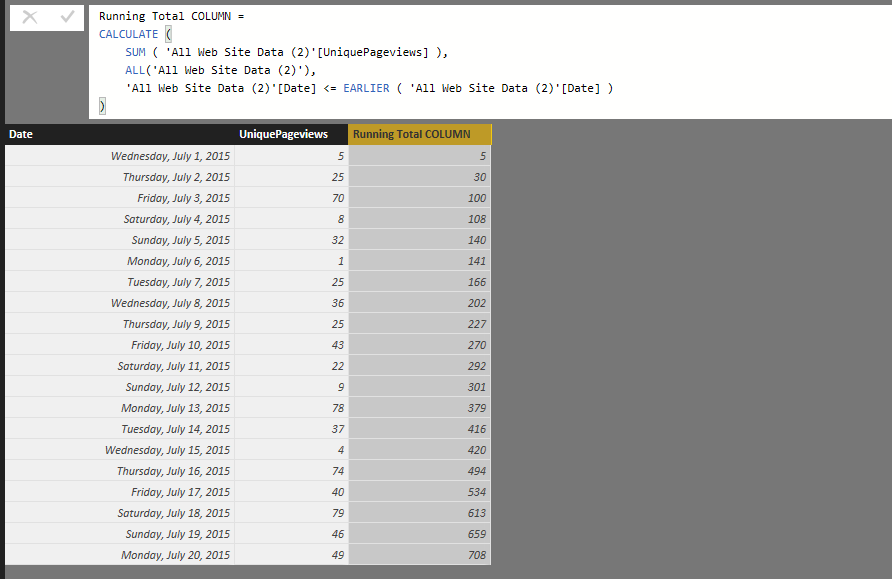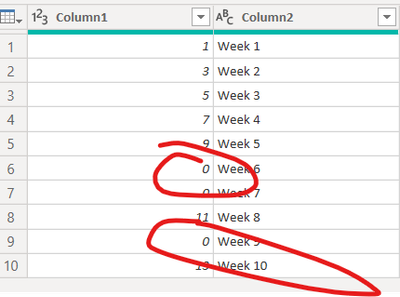cancel
Showing results for
Did you mean:Post Prodigy

## Cumulative Total

Hi,

I'm at an absolute loss as to how to calculate a cumulative total. I've tried googling, reading the forums, following the documentation, decomposing the calculation, trying it as both a measure and a calc'd column; It always seems to refer me to the same number. So for example; March will be 10, April 12, but instead of showing me 10 for March and 22 for April, it shows me 10 for March and 12 for april.

For eg: https://gyazo.com/41bd333cedac290e6980772906ff0034 with a measure

I my Month column as a date column, I've tried using all kinds of features including, calc, sum, sumx, time based functions.

The commonly reccomended filter of [Date] <= MAX [Date] always returns an error. The Earlier function returns errors with concerns there isn't a function above it. Any help would be greatly appreciated; I've spent hours today trying to work this out and I get the feeling there is a difference in Pivottable dax and powerbi dax. I want to be able to shape and transform my data in powerbi, using dax in powerbi.

Any help would be greatly appreciated.

1 ACCEPTED SOLUTIONCommunity Champion

@ElliotP Sorry about the original post. It was from my phone and had typosOkay here is the formula for Running Total as a Calculated Column (prorerly formatted)

```Running Total COLUMN =
CALCULATE (
SUM ( 'All Web Site Data (2)'[UniquePageviews] ),
ALL ( 'All Web Site Data (2)' ),
'All Web Site Data (2)'[Date] <= EARLIER ( 'All Web Site Data (2)'[Date] )
)```

And as you can see it works!And here's the MEASURE formula

```Running Total MEASURE =
CALCULATE (
SUM ( 'All Web Site Data (2)'[UniquePageviews] ),
FILTER (
ALL ( 'All Web Site Data (2)' ),
'All Web Site Data (2)'[Date] <= MAX ( 'All Web Site Data (2)'[Date] )
)
)```

Which also works...76 REPLIES 76Anonymous
Not applicable

@ElliotP

A common Measure that you’ll probably find useful in PowerPivot or SSAS Tabular Models is finding running totals.  For example, you may want to see total sales of a product as it accumulates over time, or for inventory models the total on hand at a given time.  You can find more tips and tricks at my blog, www.bipatterns.com.

Let’s start with a base measure in a very simple pivot table.
Total Sales :=
CALCULATE ( SUM ( FactSales[SalesAmount] ) )Now lets take our first attempt at computing a running total.  This is the most intuitive formula, but it has one common pitfall that isn’t necessarily easy to see right away.
Cumulative Total Sales :=
CALCULATE (
[Total Sales],
FILTER (
ALL ( DimDate[Datekey] ),
DimDate[Datekey] <= MAX ( ( DimDate[Datekey] ) )
)
)
Key parts of the Formula: The use of ALL(DimDate[DateKey]) results in the current context being ignored, so dates outside of the current pivot row context will be analyzed.  The second key step is the comparison of DimDate[Datekey] <= MAX ( ( DimDate[Datekey] ).  This means that all dates in the DateKey column that are before the current pivot table row context will be calculated.

If we put this measure on a table, we’ll get the correct numbers but we will have one issue remaining.The formula returns a number for dates that have no sales.  We need to add some error handling, which is outlined below.
Cumulative Sales (Correct) :=
IF (
COUNTROWS ( FactSales ) > 0,
CALCULATE (
[Total Sales],
FILTER (
ALL ( DimDate[Datekey] ),
DimDate[Datekey] <= MAX ( ( DimDate[Datekey] ) )
)
),
BLANK ()
)

The IF Function checks to make sure that there are sales in the current selected context, otherwise returning blank.  You can see the difference between the two measures below:If you have any questions for me, you can reach me via LinkedIn or in the PowerBI Community.

Please mark it as a solution or give a kudo if it works for you, otherwise let me know if you run into an issue and I'll do my best to assist.

Thanks,

Ryan DurkinHelper I

Hi @Anonymous , I am not able to understand how the IF statement is handling the error? Please explain in a bit more clear way. Thanks.Anonymous
Not applicable
Hi
I try the formula above but I can't get results, I don't know why. Can you help?

CALCULATE (
SUM ( vwExecucaoMensalItem[BudgetPurchaseValue] ),
FILTER (
ALL (vwExecucaoMensalItem[Date] ),
vwExecucaoMensalItem[Date] < MAX ( vwExecucaoMensalItem[Date] )
)
)Solution Sage

/sdjensenPost Prodigy

@sdjensen I have read that article and that's the base I've been working off, yet to no avail. When I either attempt to impose the measure on a table or create a new column with that data it simply gives me the same value that corresponds with the data.

Photos to better demonstrate the issue:

https://gyazo.com/ca41ce0b2d8ec572608d4afda4cffd32

https://gyazo.com/4e8d9b3e1cc38c514048272ced01a534

https://gyazo.com/14a6089654df6e90e7fd5595fd842ebd

The Date colum is set to a date, the Unique Pageviews is set to Whole Numbers. I'm honestly lost at this point.Community Champion

@ElliotP

Replace this:

All(Allwebsitedata(2) [Date]),

By

All(Allwebsitedata(2)),

The reason is because you are using the date field in your data Table, if you'll use a calendar table the formula works perfects.

Lima - PeruPost Prodigy

@Vvelarde

Thank you so much, we're making progress.

I've tried it as a measure as well as a new calculated column, yet it shows the cumulative total in each row; photos to demonstrate;

https://gyazo.com/0d365fcaaba2507bca2dffe1177837eb

https://gyazo.com/37b810f7b9f4659492b405b3362106db

As well, what do you mean in regards to date field date table. Should I set it to another table type?Community Champion

@ElliotP

For a calculated column :

CumulativeQuantity2 =
VAR CURRENTDATE='All Web Site Data (2) '[Date]
RETURN
CALCULATE(SUM('All Web Site Data (2) '[UniquePagePreviews]);FILTER(all('All Web Site Data (2)');'All Web Site Data (2) '[Date]<= CURRENTDATE))For a measure:

CumulativeQuantity-M =
CALCULATE(SUM('All Web Site Data (2) '[UniquePagePreviews]);FILTER(all('All Web Site Data (2) ');'All Web Site Data (2) '[Date]<= MAX('All Web Site Data (2) '[Date])))

Lima - PeruCommunity Champion

@ElliotP Sorry about the original post. It was from my phone and had typosOkay here is the formula for Running Total as a Calculated Column (prorerly formatted)

```Running Total COLUMN =
CALCULATE (
SUM ( 'All Web Site Data (2)'[UniquePageviews] ),
ALL ( 'All Web Site Data (2)' ),
'All Web Site Data (2)'[Date] <= EARLIER ( 'All Web Site Data (2)'[Date] )
)```

And as you can see it works!And here's the MEASURE formula

```Running Total MEASURE =
CALCULATE (
SUM ( 'All Web Site Data (2)'[UniquePageviews] ),
FILTER (
ALL ( 'All Web Site Data (2)' ),
'All Web Site Data (2)'[Date] <= MAX ( 'All Web Site Data (2)'[Date] )
)
)```

Which also works...Frequent Visitor

I had trouble with this formula, and the issue was the ALL() statement required my date column again. Shout out to this website for showing me.

https://powerbidocs.com/2020/11/08/cumulative-total-running-total-in-power-bi/

```Running Total MEASURE =
CALCULATE (
SUM ( 'All Web Site Data (2)'[UniquePageviews] ),
FILTER (
ALL ( 'All Web Site Data (2)'[Date] ),
'All Web Site Data (2)'[Date] <= MAX ( 'All Web Site Data (2)'[Date] )
)
)```Helper III

Thanks, I needed this!Anonymous
Not applicable

Is there a way to do this that keeps any filters applied to the original table? For example, if there was another filter called 'PageViewsFromCountry', for which we would like to apply a filter or slicer visual. I have tried using keep filters with no success:

``````Running Total MEASURE =
CALCULATE (
SUM ( 'All Web Site Data (2)'[UniquePageviews] ),
FILTER (
KEEPFILTERS( 'All Web Site Data (2)' ),
'All Web Site Data (2)'[Date] <= MAX ( 'All Web Site Data (2)'[Date] )
)
)``````

Edit: For anyone at this stage of their DAX journey, simply ommitting any function under FILTER in CALCULATE allows for the addition of filter conditions, preserving current context. So the cumulative total can be calculated with respect to any filters already applied to the table by:

``````Running Total MEASURE =
CALCULATE (
SUM ( 'All Web Site Data (2)'[UniquePageviews] ),
FILTER (
'All Web Site Data (2)',
'All Web Site Data (2)'[Date] <= MAX ( 'All Web Site Data (2)'[Date] )
)
)``````Regular Visitor

Doesn't work in the line chart if there are 0 values for some periodsRegular Visitor

I've followed an identical approach as you have provided here but run into this issue when it comes to the current year context and the previous year calculating the full total as opposed to just the four months of this year.Frequent Visitor

Make sure the code you are using has ALLSELECTED rather than just ALL in the filter part of the expression. This should then reflect the various slicers that you have employed on the page.

Regards

NeilRegular Visitor
Yep, I have that part in already

App Cumulative =
CALCULATE(
[# Applications],
FILTER(
ALLSELECTED( 'Applications' ),
'Applications'[APM_CREATED_DATE] <= MAX(Applications[APM_CREATED_DATE])
)
)Frequent Visitor
Hi @seancasey, here is an example I am using that works for all filter selections on related tables. I am doing my date segmentation based on a calendar table.

CumulativeInvoiceQty =
CALCULATE
SUM ( FACT_SalesOrderLines[InvoicedSOQty] ),
FILTER
(
ALLSELECTED ( 'DATE_TABLE' ),
('DATE_TABLE'[Date]) <= MAX ( DATE_TABLE[Date] )
)
)Regular Visitor

thanks @neilcotton , I think the lack of a date table is probaly what's causing me the trouble I'm having. Will add one now and see where it gets me.New Member

Hi,

Thanks for this measure as it perfectly works. However it doesn't sync with any other filter options then. For example I have a slicer and want to filter on a specific area but the chart takes all the data instead of the slicer filter. I guess it's because we're doing an All filter option in the formula. How can I fix this so that my cumulative chart also syncs with my slicer?

Regards,Resolver I

@Sean  Hi I tried using the measure and I cant get it to work. I dont have sales volumes in column format. I can only use Sales Volume as measure for the caluclation. Is there a way to make moeasure to work when using measure?

This is how the measure looks. Thank you.

Cumulative Sales volume =
CALCULATE (
SUM ( 'Actuals'[Sales Volume] ),
FILTER (
ALLSELECTED ( 'Date' ),
'Date'[Date] <= MAX ( 'Date'[Date] )
))Impactful Individual

Thank you so much for the formula!

I made an adjustment so that various filters applied in the page would work: instead of ALL(), i've used ALLSELECTED([Date]).